Question

Solve the following system using the Elimination Method; 6x+y=10 and 2x+y=12.

1.thanhcong

Hey there!

The solution to the system is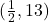To solve the system, we can multiply the second equation by -1: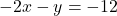Then, we add the two equations together, and solve for x: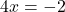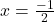Now, we plug the x value into one of the equations, and solve for y: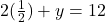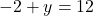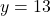Now we know that the solution to the system isHope it helps and have an amazing day!

2.RobertKer

(-1/2, 13)

Step-by-step explanation:

Multiply the 2nd equation by -1, obtaining the following system:

6x + y = 10

-2x  – y = -12

——————

Combine like terms, obtaining:

6x + y = 10

-2x  – y = -12

——————

4x     = -2

Dividing both sides by 4 yields x = -2/4, or x = -1/2

Substituting -1/2 for x in the second equation yields:

2(-1/2) + y = 12, or

-1 + y = 12.  Then y = 13, and the solution is

(-1/2, 13)